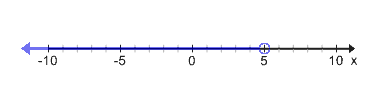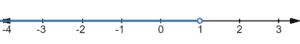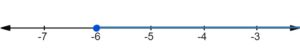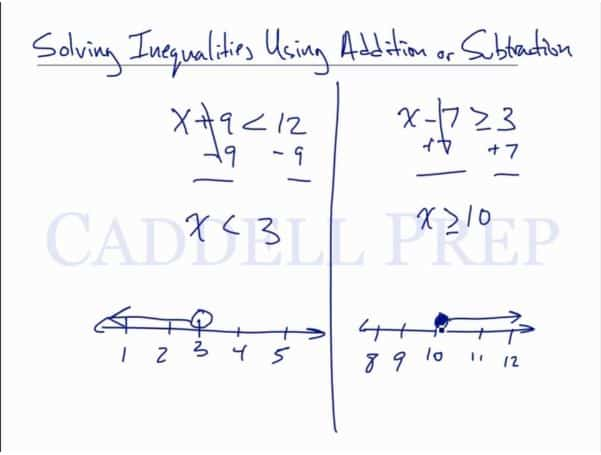In this video, we will be learning how to solve inequalities using addition or subtraction. After you finish this lesson, view all of our Algebra 1 lessons and practice problems.

Example of Solving an Inequality with Addition/Subtraction$x+7\textless12$$x+7-7\textless12-7\leftarrow$ Subtract 7 from both sides$x\textless5\leftarrow$ Graph the inequalityExample 1$x+9\textless10$

Subtract$9$ from both sides$x+9-9\textless10-9$$x\textless1$

Now, graph the inequalityExample 2$x-4\geq2$

Add$4$ from both sides$x-4+4\geq2+4$$x\geq6$

Now, graph the inequalityVideo-Lesson Transcript

Let’s go over how to solve inequalities using addition or subtraction.

Solving this is similar to a regular equation except for the inequality sign instead of an equal sign.

For example:$x + 9 \textless 12$

Let’s solve for$x$ by subtracting$9$ from both sides of the inequality.$x + 9 - 9 \textless 12 - 9$$x \textless 3$

We can graph this by drawing a number line then encircle the number$3$ then draw a line to the left which represents the numbers less than$3$.

Now, let’s take a look at subtraction.

We have$x - 7 \textgreater 3$

To solve$x$, let’s just add$7$ on both sides.$x - 7 + 7 \textgreater 3 + 7$$x \textgreater 10$

Now, let’s graph it by drawing a numbe line.

Let’s draw an open circle on$10$ and draw a line to the right to represent numbers greater than$10$.

If we change the sign of$x - 7 \textgreater 3$ into$x - 7 \geq 3$, then our answer would be$x \geq 10$.Our graph will have a solid circle on$10$ instead of an open circle. Then the line is still going to the right to represent numbers greater than$10$.